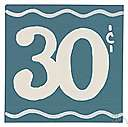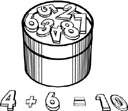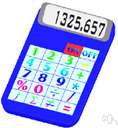# sum

Also found in: Thesaurus, Medical, Legal, Financial, Acronyms, Idioms, Encyclopedia, Wikipedia.

## sum

(sŭm)
n.
1. Mathematics
a. An amount obtained as a result of adding numbers.
b. An arithmetic problem: a child good at sums.
2. The whole amount, quantity, or number; an aggregate: the sum of the team's combined experience.
3. An amount of money: paid an enormous sum.
4. A summary: my view of the world, in sum.
5. The central idea or point; the gist.
tr.v. summed, sum·ming, sums
1. Mathematics To add.
2. To give a summary of; summarize.
Phrasal Verb:
sum up
1. To present the substance of (material) in a condensed form; summarize: sum up the day's news; concluded the lecture by summing up.
2. To describe or assess concisely: an epithet that sums up my feelings.

[Middle English summe, from Old French, from Latin summa, from feminine of summus, highest; see uper in Indo-European roots.]

## sum

(sʌm)
n
1. (Mathematics)
a. the result of the addition of numbers, quantities, objects, etc
b. the cardinality of the union of disjoint sets whose cardinalities are the given numbers
2. (Mathematics) one or more columns or rows of numbers to be added, subtracted, multiplied, or divided
3. (Mathematics) maths the limit of a series of sums of the first n terms of a converging infinite series as n tends to infinity
4. (Education) (plural) another name for number work
5. (Banking & Finance) a quantity, esp of money: he borrows enormous sums.
6. the essence or gist of a matter (esp in the phrases in sum, in sum and substance)
7. a less common word for summary
8. archaic the summit or maximum
9. (modifier) complete or final (esp in the phrase sum total)
vb, sums, summing or summed
10. (often foll by up) to add or form a total of (something)
11. (Mathematics) (tr) to calculate the sum of (the terms in a sequence)
[C13 summe, from Old French, from Latin summa the top, sum, from summus highest, from superus in a higher position; see super]

## sum

(sʊm)
n, pl sumy (ˈsʊmɪ)
(Currencies) the standard monetary unit of Uzbekistan, divided into 100 tiyin

## sum

(sʌm)

n., v. summed, sum•ming. n.
1. the aggregate of two or more numbers, magnitudes, quantities, or particulars as determined by or as if by the mathematical process of addition: The sum of 6 and 8 is 14.
2. an amount or quantity, esp. of money: to lend small sums.
3. a series of numbers or quantities to be added up.
4. an arithmetical problem to be solved, or such a problem worked out and having the various steps shown.
5. the full amount, or the whole: the sum of our knowledge.
6. the main idea, gist, or point: the sum and substance of his argument.
7. a summary.
v.t.
8. to combine into an aggregate or total (often fol. by up).
9. to ascertain the sum of, as by addition.
10. to bring into or contain in a small compass (often fol. by up).
v.i.
11. to amount.
12. sum up,
a. to express in a brief and comprehensive statement; summarize.
b. to form a quick estimate or judgment of.
Idioms:
in sum, in concise or brief form.
[1250–1300; < Latin summa sum, n. use of feminine of summus highest, superlative of superus (see superior)]
sum`ma•bil′i•ty, n.

## sum-

var. of sub- before m: summon.

## sum

(sŭm)
The result of adding numbers or quantities. The sum of 6 and 9, for example, is 15, and the sum of 4x and 5x is 9x.

## Sum

a quantity of money; a number of things; a host; an assembly.
Examples: sum of conceits, 1576; of facts, 1840; of gold, 1375; of happiness, 1772; of malt, 1528; of men, 1450; of misery, 1827; of silver, 1596; of soldiers, 1400; of Muscovado sugar, 1680; of tobacco, 1872; of treasure, 1300.

## sum

Past participle: summed
Gerund: summing

Imperative
sum
sum
Present
I sum
you sum
he/she/it sums
we sum
you sum
they sum
Preterite
I summed
you summed
he/she/it summed
we summed
you summed
they summed
Present Continuous
I am summing
you are summing
he/she/it is summing
we are summing
you are summing
they are summing
Present Perfect
I have summed
you have summed
he/she/it has summed
we have summed
you have summed
they have summed
Past Continuous
I was summing
you were summing
he/she/it was summing
we were summing
you were summing
they were summing
Past Perfect
Future
I will sum
you will sum
he/she/it will sum
we will sum
you will sum
they will sum
Future Perfect
I will have summed
you will have summed
he/she/it will have summed
we will have summed
you will have summed
they will have summed
Future Continuous
I will be summing
you will be summing
he/she/it will be summing
we will be summing
you will be summing
they will be summing
Present Perfect Continuous
I have been summing
you have been summing
he/she/it has been summing
we have been summing
you have been summing
they have been summing
Future Perfect Continuous
I will have been summing
you will have been summing
he/she/it will have been summing
we will have been summing
you will have been summing
they will have been summing
Past Perfect Continuous
I had been summing
you had been summing
he/she/it had been summing
we had been summing
you had been summing
they had been summing
Conditional
I would sum
you would sum
he/she/it would sum
we would sum
you would sum
they would sum
Past Conditional
I would have summed
you would have summed
he/she/it would have summed
we would have summed
you would have summed
they would have summed
ThesaurusAntonymsRelated WordsSynonymsLegend:
 Noun 1sum - a quantity of money; "he borrowed a large sum"; "the amount he had in cash was insufficient"gain - the amount by which the revenue of a business exceeds its cost of operatingreceipts, revenue, gross - the entire amount of income before any deductions are madecash surrender value - the amount that the insurance company will pay on a given life insurance policy if the policy is cancelled prior to the death of the insuredcontribution - an amount of money contributed; "he expected his contribution to be repaid with interest"deductible - (taxes) an amount that can be deducted (especially for the purposes of calculating income tax)defalcation - the sum of money that is misappropriatedred ink, red, loss - the amount by which the cost of a business exceeds its revenue; "the company operated at a loss last year"; "the company operated in the red last year"assets - anything of material value or usefulness that is owned by a person or companyfigure - an amount of money expressed numerically; "a figure of \$17 was suggested"coverage, insurance coverage - the total amount and type of insurance carriedcash advance, advance - an amount paid before it is earnedpaysheet, payroll - the total amount of money paid in wages; "the company had a large payroll"peanuts - an insignificant sum of money; a trifling amount; "her salary is peanuts compared to his"purse - a sum of money offered as a prize; "the purse barely covered the winner's expenses"purse - a sum of money spoken of as the contents of a money purse; "he made the contribution out of his own purse"; "he and his wife shared a common purse" 2sum - a quantity obtained by the addition of a group of numbersquantity - the concept that something has a magnitude and can be represented in mathematical expressions by a constant or a variablegrand total - the sum of the sums of several groups of numberssubtotal - the sum of part of a group of numbers 3 sum - the final aggregate; "the sum of all our troubles did not equal the misery they suffered"aggregation, collection, accumulation, assemblage - several things grouped together or considered as a wholecongeries, conglomeration, aggregate - a sum total of many heterogenous things taken together 4 sum - the choicest or most essential or most vital part of some idea or experience; "the gist of the prosecutor's argument"; "the heart and soul of the Republican Party"; "the nub of the story"cognitive content, mental object, content - the sum or range of what has been perceived, discovered, or learnedbare bones - (plural) the most basic facts or elements; "he told us only the bare bones of the story"hypostasis - (metaphysics) essential nature or underlying realityhaecceity, quiddity - the essence that makes something the kind of thing it is and makes it different from any otherquintessence - the purest and most concentrated essence of somethingstuff - a critically important or characteristic component; "suspense is the very stuff of narrative" 5sum - the whole amount      whole, unit - an assemblage of parts that is regarded as a single entity; "how big is that part compared to the whole?"; "the team is a unit" 6 sum - a set containing all and only the members of two or more given sets; "let C be the union of the sets A and B"set - a group of things of the same kind that belong together and are so used; "a set of books"; "a set of golf clubs"; "a set of teeth"direct sum - a union of two disjoint sets in which every element is the sum of an element from each of the disjoint sets Verb 1 sum - be a summary of; "The abstract summarizes the main ideas in the paper"sum up, summarize, summarise, resume - give a summary (of); "he summed up his results"; "I will now summarize"say, state, tell - express in words; "He said that he wanted to marry her"; "tell me what is bothering you"; "state your opinion"; "state your name" 2sum - determine the sum of; "Add all the people in this town to those of the neighboring town"add together, add - make an addition by combining numbers; "Add 27 and 49, please!"count, numerate, enumerate, number - determine the number or amount of; "Can you count the books on your shelf?"; "Count your change"

## sum

noun
1. Large sums of money were lost.
2. calculation, figures, arithmetic, problem, numbers, reckonings, mathematics, maths (Brit. informal), tally, math (U.S. informal), arithmetical problem I can't do my sums.
3. The sum of all the angles of a triangle is 180 degrees.
4. totality, whole, aggregate, entirety, beginning and end, summation The sum of evidence points to the crime resting on them.
in sum It is a situation, in sum, devoid of logic.
sum something or someone up size up, estimate (informal), get the measure of, form an opinion of My mother probably summed her up better than I ever could.
sum something up summarize, express concisely, express pithily, express in a word He summed his weakness up in one word: 'Disastrous.'
sum up summarize, review, recapitulate, close, conclude, put something in a nutshell When the judge summed up it was clear he wanted a guilty verdict.

## sum

noun
1. A number or quantity obtained as a result of addition:
Archaic: tale.
2. An amount or quantity from which nothing is left out or held back:
Informal: work (used in plural).
Idioms: everything but the kitchen sink, lock, stock, and barrel, the whole ball of wax.
3. An organized array of individual elements and parts forming and working as a unit:
4. A condensation of the essential or main points of something:
Informal: recap.
verb
To combine (figures) to form a sum.Also used with up:
add (up), cast, foot (up), tot (up), total, totalize.
phrasal verb
sum up
To give a recapitulation of the salient facts of:
Informal: recap.
Translations
كَمِّيَّهمَبْلَغمَجموعمَسْأَلَةٌ حِسَابِيَّةمَسْأَلَه حِسابِيَّه
součetsumavýpočetobnospočty
sumbeløbregnestykke
zbroj
számtanpélda
reikningsdæmi; samlagningardæmisummaupphæî

합계
aritmetikos skaičiavimasaritmetikos uždavinysbendra suma
matemātikas uzdevumssumma
počty
povzetiseštevekvsota
การคำนวณผลรวม
tổngtổng số

## sum

[sʌm] N
1. (= piece of arithmetic) → suma f, adición f
I was very bad at sumsera muy malo en aritmética
to do one's sumshacer cuentas
to do sums in one's head
2. (= total) → suma f, total m; (= amount of money) → suma f, importe m
in sum
more/greater than the sum of its parts
sum totaltotal m (completo)
the sum total of my ambitions isla meta de mis ambiciones es ..., lo único que ambiciono es ...
that was the sum (total) of his achievementsy de allí no pasó
sum up
A. VI + ADV (= summarize) → resumir; [judge] → recapitular
to sum up, I would sayen resumidas cuentas, yo diría
B. VT + ADV
1. (= summarize) [+ speech, facts, argument] → resumir
you could sum up what he said in a couple of wordsse podría resumir lo que dijo en dos palabras
to sum up an argument
2. (= encapsulate) → resumir
that picture summed up the situation for meesa fotografía resumió la situación para mí or captaba la situación en un solo trazo
3. (= assess) [+ person] → calar; [+ situation] → evaluar
they had summed him up and liked what they foundlo habían calado y les había agradado lo que descubrieron
he summed up the situation quickly

## sum

[ˈsʊm] n
[money] →
Large sums of money were lost → De grosses sommes d'argents furent perdues.
(= calculation) →
She's good at sums → Elle est bonne en calcul.
(= total of added numbers) →
The sum of the angles of a triangle is 180 degrees → La somme de les angles d'un triangle fait 180 degrés.
(= total) [achievements, experience, evidence] →
sum up
vt sep
(= summarize) → résumer
(= size up) [+ person, situation] → cerner
virésumer

## sum

n
(= total)Summe f; that was the sum (total) of his achievementsdas war alles, was er geschafft hatte; the sum total of my ambitionsdas Ziel meiner Wünsche
(of money)Betrag m, → Summe f
(esp Brit: = calculation) → Rechenaufgabe f; to do sums (in one’s head)(im Kopf) rechnen; I was bad at sumsich war schlecht im Rechnen
(form: = essence) in summit einem Wort, zusammengefasst

## sum

[sʌm] n (piece of arithmetic) → ; (amount of money) → somma
the sum of 6 and 4 is 10 → 6 più 4 fa 10
that is the sum (total) of his achievements → questo è tutto quello che ha fatto
sum up
1. vt + adv (review) → ; (evaluate rapidly) →
to sum up an argument →
she quickly summed him up →
he summed up the situation quickly →
2. vi + advriassumere
to sum up ... → per riassumere..., riassumendo...

## sum

(sam) noun
1. the amount or total made by two or more things or numbers added together. The sum of 12, 24, 7 and 11 is 54.
2. a quantity of money. It will cost an enormous sum to repair the swimming pool.
3. a problem in arithmetic. My children are better at sums than I am.
sum total
the complete or final total. The sum total of the damage cannot be calculated.
sum uppast tense, past participle summed verb
to give the main or important points of. He summed up the various proposals.

## sum

obnos, výpočet sum laskutehtävä, summa zbroj 合計, 計算 합계 summa การคำนวณ, ผลรวม tổng, tổng số , 总和
References in classic literature ?
The sum required was far too great for any individual, or even any single State, to provide the requisite millions.
Here the speaker sat down in his place, And directed the Judge to refer to his notes And briefly to sum up the case.
At what sum do you estimate this bank's proportion of the country's loss by me?
He had fixed on that number because forty-three was the sum of his and Sonya's joint ages.
AN OLD WOMAN having lost the use of her eyes, called in a Physician to heal them, and made this bargain with him in the presence of witnesses: that if he should cure her blindness, he should receive from her a sum of money; but if her infirmity remained, she should give him nothing.
Godfrey Ablewhite was entrusted with the care of a sum of twenty thousand pounds--as one of two Trustees for a young gentleman, who was still a minor in the year eighteen hundred and forty-eight.
This violent resolution was not lasting; his zeal gave way to his avarice, and he could not think of losing so large a sum as he knew he might expect for our ransom: he therefore sent us word that it was in our choice either to die, or to pay him thirty thousand crowns, and demanded to know our determination.
Night found him, not only equipped with money of his own, but once more (as on that former occasion) saddled with a considerable sum of other people's.
He did not stipulate for any particular sum, my dear Fanny; he only requested me, in general terms, to assist them, and make their situation more comfortable than it was in his power to do.
I do not remember how I got the money to buy them; to be sure it was no great sum; but it must have been given me out of the sums we were all working so hard to make up for the debt, and the interest on the debt (that is always the wicked pinch for the debtor
cried Colbert, struck, as Fouquet had been, with the generosity of the sum for a soldier's pension, "why, that would be a pension of twenty thousand livres?
You consider it, undoubtedly, a handsome sum of money.

Site: Follow: Share:
Open / Close